Data Science using Python & R Programing

Venue : RND Consultancy Services, Serampore, Chatra, Hooghly, WB. Phone : +919830546476 / +918240819346

After completion of this course student will get an overview about what is data science today and how they can implement python and R language for data science.

Basic knowledge of C or Java and basic python and statistics.

Introduction to Python for Data Science

Python DataScience

Python Pandas

Python NumPy

Python Scipy

Python Matplotlib

Python Data Processing

Python Data Operation

Data cleansing

Processing CSV Data

Python relational Database

Python Data Visualization

Python chart properties

Chart Styling

Python Graph Data

Python Scatter plot

Python 3D charts

Statistical Data Analysis

Measuring central tendency

Measuring variance

Normal , binomial and Poisson distribution

Correlation and linear regression

Introduction To R

Overview of R language

DataType ,variable and operators

Decision Making and loops

Function

Array

Factors

DataFrame

Package

CSV files

Excel file

Binary files

Database

R-pie charts

R- Bar charts

Histogram

Line graph

Scatter diagram

R Statistics

Mean , median and mode

Regression

Normal , Binomial and Poisson distribution

Analysis of covariance

Time series analysis

Q & A

FIND US

Serampore, Chatra, Hooghly, West Bengal, India.
Phone: +919830546476 / +918240819346

E-mail: rndconsultancyservice@gmail.com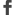Facebook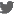Twitter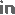LinkedInGoogle+Flicker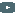YoutubePinterest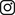Instagram